## Monday, January 08, 2007

### A Post Keynesian Model of Growth and Distribution (Part 3 of 4)

2.5 The Valuation Ratio, Dividends, Capital Gains, and the Interest Rate

The previous part describes the corporate sector in a model of steady-state growth. The first part introduces the model. This part extends the model to consider how financial institutions mediate household ownership of corporations.

The value of the capital goods owned by corporations is K. Households do not own these capital goods. Rather, they own stock. The market value of stock is (v K). The ratio of the market value of stock to the value of the capital goods used by corporations (the "book value") is known as the valuation ratio, v. I assume that the valuation ratio is constant along a steady-state growth path; variations in the valuation ration reflect short-term speculation.

The steady-state valuation ratio cannot be below unity. Otherwise, corporations would expand by purchasing financial assets, rather than capital goods. Since households typically do not buy blast-furnaces and other real capital goods, the valuation ratio can exceed unity.

Profits not retained by the corporate sector are paid out to households in the form of dividends. By assumption, dividends are (1 - sc)P. The increase in the value at the end of a year of the capital goods owned by corporations is net investment, I. The increase in the value of all shares is v I, while the value of new shares sold is f I. This latter quantity is needed to finance that portion of net investment not covered by retained profits. The difference in these quantities (v - f) I, is the value of capital gains.

Interest payments on financial capital are such that one consume them entirely and leave one's capital value unaltered. Thus, interest payments in this model are equivalent to the sum of dividends and capital gains. The rate of interest, i, is then defined by Equation 23: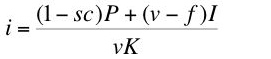(23)
Some algebraic manipulations and the definitions of the rate of profits and the rate of growth given by Equations 3 and 2, respectively, yield Equation 24: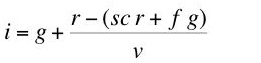(24)
Substituting from Equation 8, I obtain: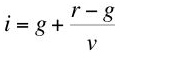(25)
Or:(26)
Assume that the rate of interest is greater than the rate of growth. Then, a valuation ratio greater than unity is equivalent to the rate of profits on industrial capital exceeding the rate of interest on financial capital along a steady state growth path.

2.6 Savings Decisions and Increases in Wealth

I assume the existence of a class of workers and a class of pure capitalists (rentiers) in the steady state. Members of both classes save, and therefore both obtain a share of profits. The rentiers obtain all their income from profits.

If both classes are to persist, the wealth of each class must grow at the steady-state growth rate, g. Equation 27 equates the rate of growth o rentier wealth: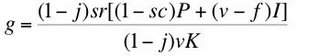(27)
where sr is the capitalists' (marginal and average) savings propensity and j is the proportion of stock owned by the workers. Equation 28 equates the steady-state rate of growth and the rate of growth of workers' wealth: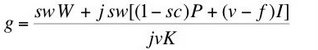(28)
where sw is the workers' (marginal and average) savings propensity.

Equations 27 and 28 determine the valuation ratio and the proportion of stock owned by the workers. Some variables in these two equations have already been determined: wages, profits, net investment, and the value of capital. The rate of growth, the proportion of net investment financed by additional stock, the retention ratio, and the workers' and the capitalists' savings propensities of the model.

2.7 Parameter Ranges

Certain conditions must be met for a steady-state growth path to exist in the above model. Given a positive price of steel, Equation 29 follows from Equation 11: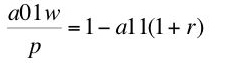(29)
Then the wage is positive if and only if: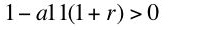(30)
Or: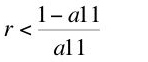(31)
By similar reasoning based on Equation 19, outputs of corn and steel are positive if and only if the inequality in Display 32 holds: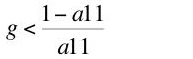(32)
Displays 31 and 32 show a limit on the rate of profits and on the rate of growth. This limit is imposed by a parameter of the chosen technique. The inequality in Display 33 must hold for the rate of profits and the rate of growth to be positive:(33)

The model imposes a necessary condition on savings propensities. Define sh as a weighted savings propensities over households: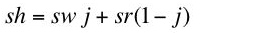(34)
Table 1 uses the household savings propensity to show the sources of savings. The first three rows show savings out of profits, while the last row shows savings from wages. Since intended savings equals investment along a steady-state path, Equation 35 must hold: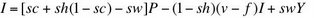(35)
Or: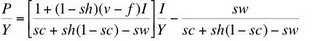(36)
I assume both profit and wage shares are positive: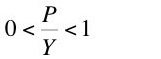(37)
Display 38 gives a necessary condition to ensure positive shares for both classes: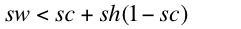(38)

This condition is that the savings rate out of wages be less than the sum of the proportion of retained earnings and the savings rate out of profits distributed as dividends.
 Savings From Dividends sh(1 - sc)P Savings From Retained Earnings sc P Minus Consumption From Capital Gains - (1 - sh)(v - f)I Savings From Wages sw(Y - P)